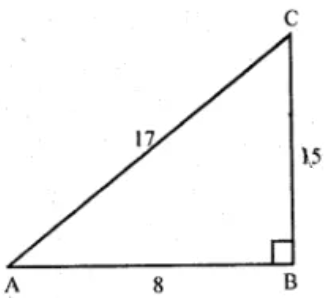Guru

# If A is an acute angle and sec A = 17/8, find all other trigonometric ratios of angle A (using trigonometric identities).

• 1

Book- ML Aggarwal
Board- ICSE
Publication- Avichal
Chapter- Trigonometric Identities

If A is an acute angle and sec A = 17/8, find all other trigonometric ratios of angle A (using trigonometric identities).

This is important ques..  Pls provide solution with figure

ML Aggarwal, ques no 2

Share

1. Solution:Given that

sec A = 17/8 and A is an acute angle

So, in ∆ ABC we have ∠B = 90o

And,

AC = 17 and AB = 8

By Pythagoras theorem,

BC = √(AC2 – AB2)

= √(172 – 82) = √(289 – 64) = √225

= 15

Now,

sin A = BC/AC = 15/17

cos A = 1/sec A = 8/17

tan A = BC/AB = 15/8

cot A = 1/tan A= 8/15

cosec A = 1/sin A = 17/15

• 0